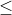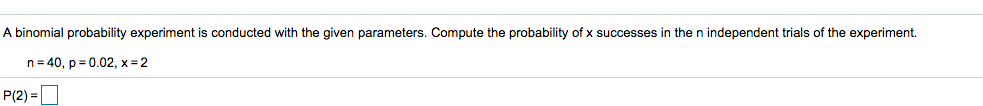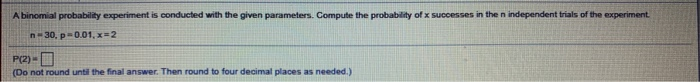Question

# A binomial probability experiment is conducted with the given parameters. Compute the probability of x successes...

A binomial probability experiment is conducted with the given parameters. Compute the probability of x successes in the n independent trials of the experiment.

n=12​

p=0.35​

x less than or equals 4

Solution

Given that ,

Using binomial probability formula ,

P(X = x) = (n C x) * px * (1 - p)n - x

P(X4) = P(X = 0) + P(X = 1) + P(X = 2) + P(X = 3) + P(X = 4)

= (12 C 0) * 0.350 * (0.65)12 + (12 C 1) * 0.351 * (0.65)11 + (12 C 2) * 0.352 * (0.65)10 +

(12 C 3) * 0.353 * (0.65)9 + (12 C 4) * 0.354 * (0.65)8

= 0.5833

Probability = 0.5833

#### Earn Coins

Coins can be redeemed for fabulous gifts.

Similar Homework Help Questions
• ### A binomial probability experiment is conducted with the given parameters. Compute the probability of x successes...

A binomial probability experiment is conducted with the given parameters. Compute the probability of x successes in the n independent trials of the experiment. n equals 13n=13​, p equals 0.25p=0.25​, x less than or equals 4x≤4 The probability of x less than or equals 4x≤4 successes is

• ### A binomial probability experiment is conducted with the given parameters. Compute the probability of x successes...

A binomial probability experiment is conducted with the given parameters. Compute the probability of x successes in the n independent trials of the experiment. n equals 9​, p equals 0.9​, x less than or equals 3

• ### The binomial probability experiment is conducted with the given parameters. Compute the probability of x successes in t...

The binomial probability experiment is conducted with the given parameters. Compute the probability of x successes in the n independent trials of the experiment.N=14, p=0.55, x≤4The probability of obtaining x successes in n independent trials of a binomial experiment is given byP(x)=nCxpx(1-p)n-x,  x=0,1,2,……where p is the probability of success.

• ### A binomial probability experiment is conducted with the given parameters. Compute the probability of x successes...

A binomial probability experiment is conducted with the given parameters. Compute the probability of x successes in the n independent trials of the experiment. n = 10, p = 0.1, x < or = to 4

• ### A binomial probability experiment is conducted with the given parameters. Compute the probability of x successes...

A binomial probability experiment is conducted with the given parameters. Compute the probability of x successes in the n independent trials of the experiment. n=10 , p=0.45 , x=2

• ### A binomial probability experiment is conducted with the given parameters. Compute the probability of x successes...

A binomial probability experiment is conducted with the given parameters. Compute the probability of x successes in the n independent trials of the experiment. n = 20, p = 0.8, x = 10

• ### a binomial probability experiment is conducted with the given parameters. compute the probability of x successes...

a binomial probability experiment is conducted with the given parameters. compute the probability of x successes in the n independent trials of the experiment. n=10, p=0.8,x=7. P(7)=

• ### A binomial probability experiment is conducted with the given parameters. Compute the probability of x successes...A binomial probability experiment is conducted with the given parameters. Compute the probability of x successes in the n independent trials of the experiment. n = 40, p = 0.02, x-2 P(2)

• ### A binomial probability experiment is conducted with the given parameters. Compute the probability of successes in...A binomial probability experiment is conducted with the given parameters. Compute the probability of successes in the n independent trials of the experiment n-30, p=0.01, x=2 P(2)- (Do not round until the final answer. Then round to four decimal places as needed.)

• ### A binomial probability experiment is conducted with the given parameters. Compute the probability of successes in...

A binomial probability experiment is conducted with the given parameters. Compute the probability of successes in the n independent trials of the experiment n=10, p=0.6.x=9 P(9) = _______  (Do not round until the final answer. Then round to four decimal places as needed.)